An ideal gas of mass m in a state A goes to another state B via three different processes as shown in figure. If Q1, Q2 and Q3 denote the heat absorbed by the gas along the three paths, then -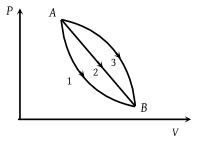(1) Q1 < Q2 < Q3

(2) Q1 < Q2 = Q3

(3) Q1 = Q2 > Q3

(4) Q1 > Q2 > Q3

Concept Questions :-

First law of thermodynamics
High Yielding Test Series + Question Bank - NEET 2020

Difficulty Level:

Which of the following graphs correctly represents the variation of $\beta =-\left(dV/dP\right)/V$ with P for an ideal gas at constant temperature

(1)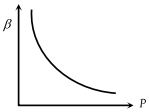(2)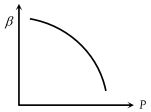(3)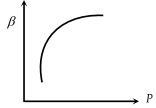(4)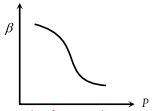Concept Questions :-

Types of processes
High Yielding Test Series + Question Bank - NEET 2020

Difficulty Level:

A thermodynamic process is shown in the figure. The pressures and volumes corresponding to some points in the figure are : ${P}_{A}=3×{10}^{4}Pa,\text{\hspace{0.17em}}{P}_{B}=8×{10}^{4}Pa$ and ${V}_{A}=2×{10}^{-3}{m}^{3},\text{\hspace{0.17em}}{V}_{D}=5×{10}^{-3}{m}^{3}$. In process AB, 600 J of heat is added to the system and in process BC, 200 J of heat is added to the system. The change in internal energy of the system in process AC would be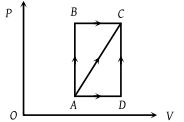(1) 560 J

(2) 800 J

(3) 600 J

(4) 640 J

Concept Questions :-

First law of thermodynamics
High Yielding Test Series + Question Bank - NEET 2020

Difficulty Level:

P-V plots for two gases during adiabatic process are shown in the figure. Plots 1 and 2 should correspond respectively to -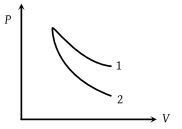(1) He and O2

(2) O2 and He

(3) He and Ar

(4) O2 and N2

Concept Questions :-

Types of processes
High Yielding Test Series + Question Bank - NEET 2020

Difficulty Level:

Four curves A, B, C and D are drawn in the adjoining figure for a given amount of gas. The curves which represent adiabatic and isothermal changes are -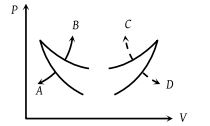(1) C and D respectively

(2) D and C respectively

(3) A and B respectively

(4) B and A respectively

Concept Questions :-

Types of processes
High Yielding Test Series + Question Bank - NEET 2020

Difficulty Level:

In pressure-volume diagram given below, the isochoric, isothermal, and isobaric parts respectively are -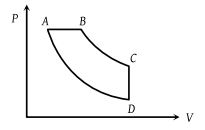(2) DC, CB, DA

(3) AB, BC, CD

(4) CD, DA, AB

Concept Questions :-

Types of processes
High Yielding Test Series + Question Bank - NEET 2020

Difficulty Level:

An ideal gas is taken through the cycle ABCA, as shown in the figure. If the net heat supplied to the gas in the cycle is 5 J, the work done by the gas in the process CA is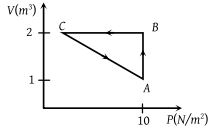(1) – 5 J

(2) – 10 J

(3) – 15 J

(4) – 20 J

Concept Questions :-

Cyclic process
High Yielding Test Series + Question Bank - NEET 2020

Difficulty Level:

In the following indicator diagram, the net amount of work done will be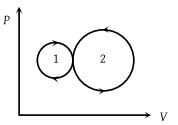(1) Positive

(2) Negative

(3) Zero

(4) Infinity

High Yielding Test Series + Question Bank - NEET 2020

Difficulty Level:

A cyclic process for 1 mole of an ideal gas is shown in figure in the V-T, diagram. The work done in AB, BC and CA respectively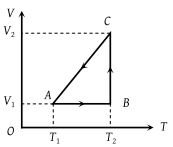(1) $0,\text{\hspace{0.17em}}R{T}_{2}\mathrm{ln}\left(\frac{{V}_{1}}{{V}_{2}}\right)\text{\hspace{0.17em}},\text{\hspace{0.17em}}R\text{\hspace{0.17em}}\left({T}_{1}-{T}_{2}\right)$

(2) $R\left({T}_{1}-{T}_{2}\right),\text{\hspace{0.17em}}0,\text{\hspace{0.17em}}R{T}_{1}\mathrm{ln}\frac{{V}_{1}}{{V}_{2}}$

(3) $0,\text{\hspace{0.17em}}R{T}_{2}\mathrm{ln}\left(\frac{{V}_{2}}{{V}_{1}}\right)\text{\hspace{0.17em}},\text{\hspace{0.17em}}R\text{\hspace{0.17em}}\left({T}_{1}-{T}_{2}\right)$

(4) $0,\text{\hspace{0.17em}}R{T}_{2}\mathrm{ln}\left(\frac{{V}_{2}}{{V}_{1}}\right)\text{\hspace{0.17em}},\text{\hspace{0.17em}}R\text{\hspace{0.17em}}\left({T}_{2}-{T}_{1}\right)$

High Yielding Test Series + Question Bank - NEET 2020

Difficulty Level:

A cyclic process ABCD is shown in the P-V diagram. Which of the following P-T curves represent the same process ?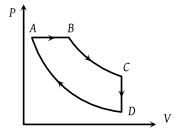(1)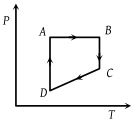(2)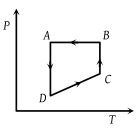(3)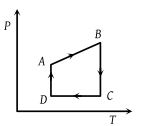(4)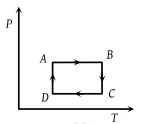Concept Questions :-

Types of processes